Скачать презентацию Ternopil National Economic University American-Ukrainian School of Computer

Topic 13__Tree.pptx

• Количество слайдов: 22Ternopil National Economic University American-Ukrainian School of Computer Science Algorithmization and Programming Topic 13: Tree Dr. Ihor Paliy Assistant Professor, Director of American-Ukrainian School for Computer Science Email: [email protected] edu. ua Web: www. umcs. maine. edu/~aus Ternopil, 2012Outline 2 Tree Overview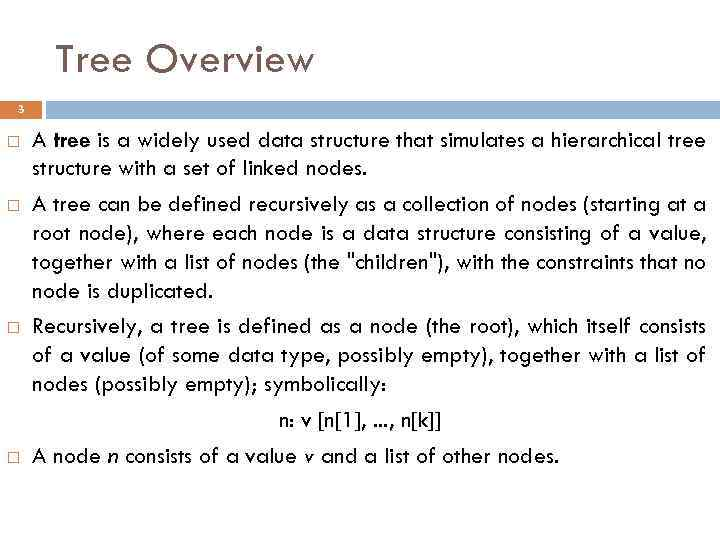Tree Overview 3 A tree is a widely used data structure that simulates a hierarchical tree structure with a set of linked nodes. A tree can be defined recursively as a collection of nodes (starting at a root node), where each node is a data structure consisting of a value, together with a list of nodes (the "children"), with the constraints that no node is duplicated. Recursively, a tree is defined as a node (the root), which itself consists of a value (of some data type, possibly empty), together with a list of nodes (possibly empty); symbolically: n: v [n, . . . , n[k]] A node n consists of a value v and a list of other nodes.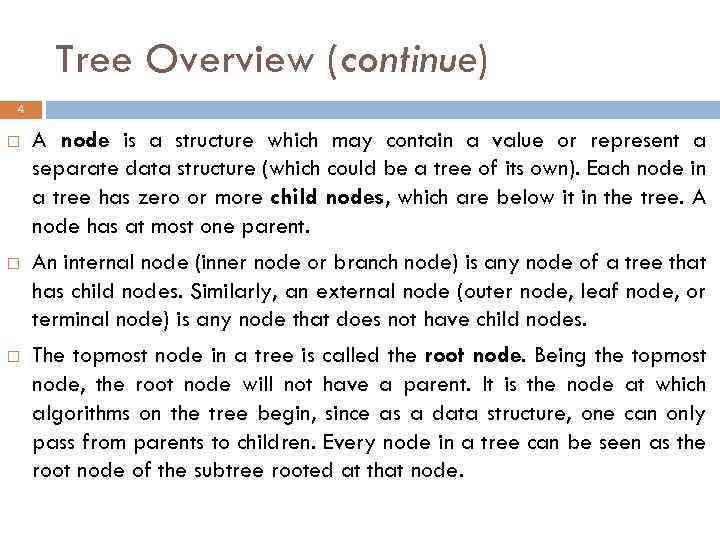Tree Overview (continue) 4 A node is a structure which may contain a value or represent a separate data structure (which could be a tree of its own). Each node in a tree has zero or more child nodes, which are below it in the tree. A node has at most one parent. An internal node (inner node or branch node) is any node of a tree that has child nodes. Similarly, an external node (outer node, leaf node, or terminal node) is any node that does not have child nodes. The topmost node in a tree is called the root node. Being the topmost node, the root node will not have a parent. It is the node at which algorithms on the tree begin, since as a data structure, one can only pass from parents to children. Every node in a tree can be seen as the root node of the subtree rooted at that node.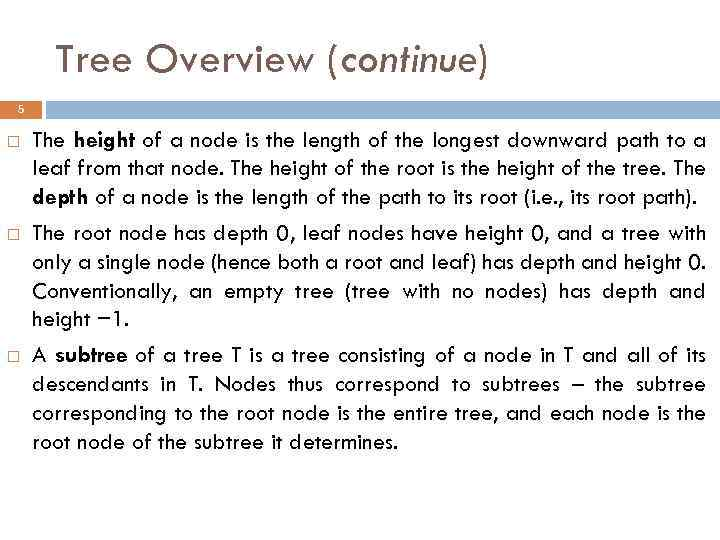Tree Overview (continue) 5 The height of a node is the length of the longest downward path to a leaf from that node. The height of the root is the height of the tree. The depth of a node is the length of the path to its root (i. e. , its root path). The root node has depth 0, leaf nodes have height 0, and a tree with only a single node (hence both a root and leaf) has depth and height 0. Conventionally, an empty tree (tree with no nodes) has depth and height − 1. A subtree of a tree T is a tree consisting of a node in T and all of its descendants in T. Nodes thus correspond to subtrees – the subtree corresponding to the root node is the entire tree, and each node is the root node of the subtree it determines.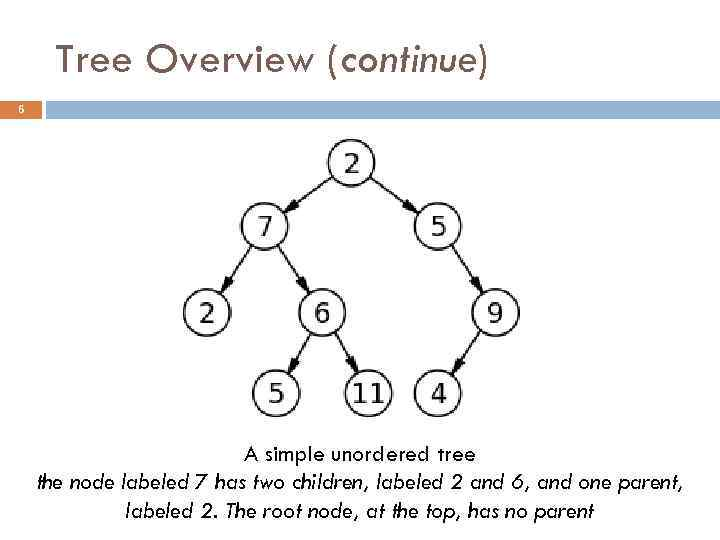Tree Overview (continue) 6 A simple unordered tree the node labeled 7 has two children, labeled 2 and 6, and one parent, labeled 2. The root node, at the top, has no parent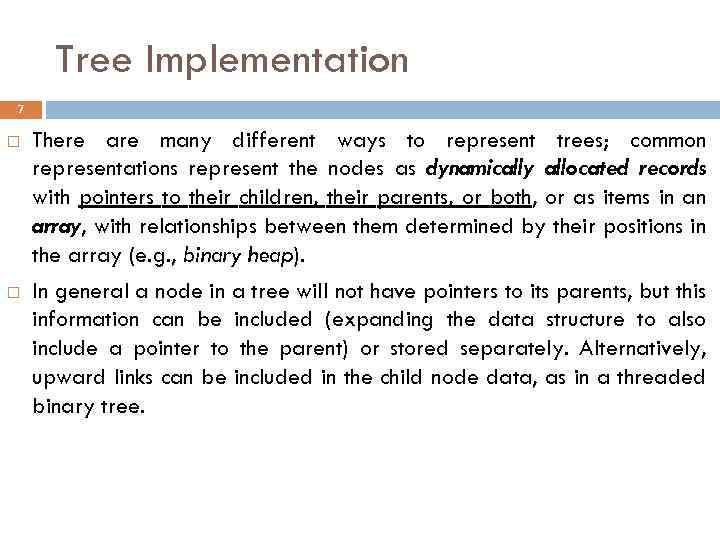Tree Implementation 7 There are many different ways to represent trees; common representations represent the nodes as dynamically allocated records with pointers to their children, their parents, or both, or as items in an array, with relationships between them determined by their positions in the array (e. g. , binary heap). In general a node in a tree will not have pointers to its parents, but this information can be included (expanding the data structure to also include a pointer to the parent) or stored separately. Alternatively, upward links can be included in the child node data, as in a threaded binary tree.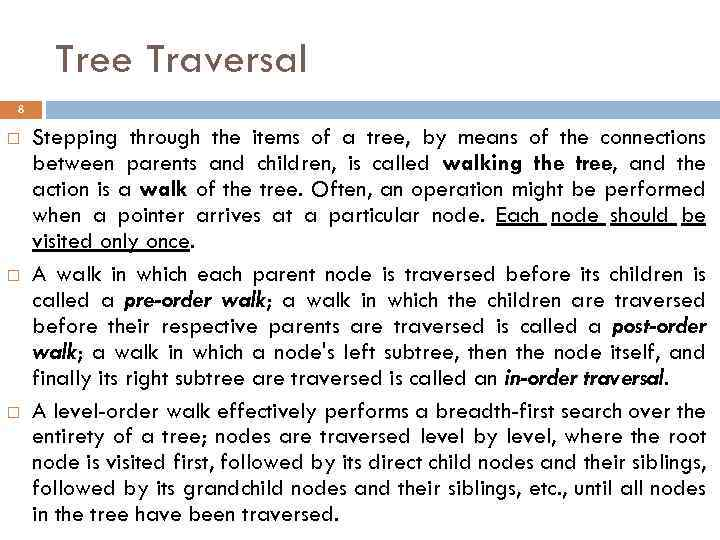Tree Traversal 8 Stepping through the items of a tree, by means of the connections between parents and children, is called walking the tree, and the action is a walk of the tree. Often, an operation might be performed when a pointer arrives at a particular node. Each node should be visited only once. A walk in which each parent node is traversed before its children is called a pre-order walk; a walk in which the children are traversed before their respective parents are traversed is called a post-order walk; a walk in which a node's left subtree, then the node itself, and finally its right subtree are traversed is called an in-order traversal. A level-order walk effectively performs a breadth-first search over the entirety of a tree; nodes are traversed level by level, where the root node is visited first, followed by its direct child nodes and their siblings, followed by its grandchild nodes and their siblings, etc. , until all nodes in the tree have been traversed.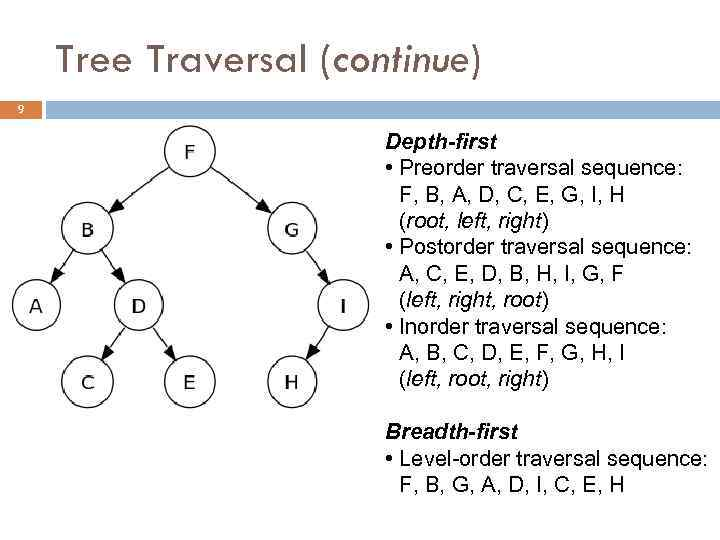Tree Traversal (continue) 9 Depth-first • Preorder traversal sequence: F, B, A, D, C, E, G, I, H (root, left, right) • Postorder traversal sequence: A, C, E, D, B, H, I, G, F (left, right, root) • Inorder traversal sequence: A, B, C, D, E, F, G, H, I (left, root, right) Breadth-first • Level-order traversal sequence: F, B, G, A, D, I, C, E, HTree Traversal (continue) 10 Preorder preorder(node) if node == null then return visit(node) preorder(node. left) preorder(node. right) Postorder recursive. Postorder(node) if node == null then return recursive. Postorder(node. left) recursive. Postorder(node. right) visit(node)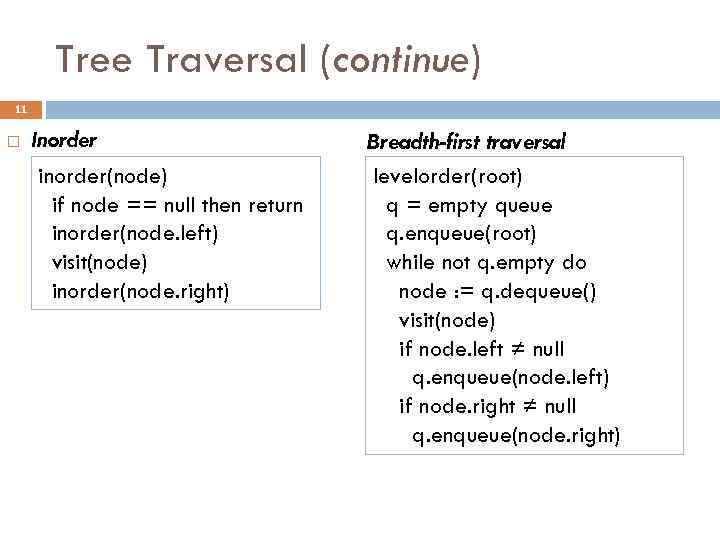Tree Traversal (continue) 11 Inorder inorder(node) if node == null then return inorder(node. left) visit(node) inorder(node. right) Breadth-first traversal levelorder(root) q = empty queue q. enqueue(root) while not q. empty do node : = q. dequeue() visit(node) if node. left ≠ null q. enqueue(node. left) if node. right ≠ null q. enqueue(node. right)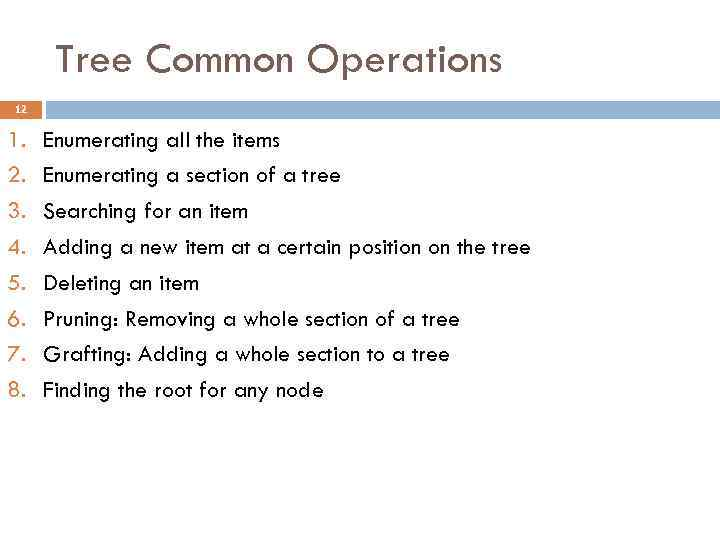Tree Common Operations 12 1. 2. 3. 4. 5. 6. 7. 8. Enumerating all the items Enumerating a section of a tree Searching for an item Adding a new item at a certain position on the tree Deleting an item Pruning: Removing a whole section of a tree Grafting: Adding a whole section to a tree Finding the root for any node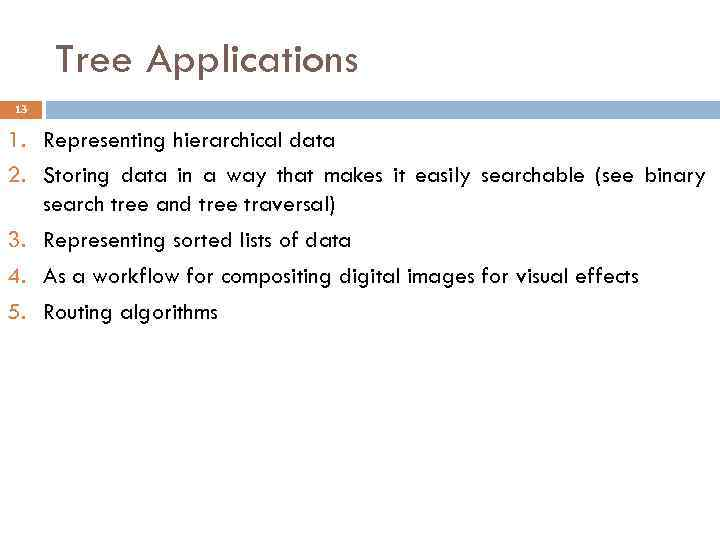Tree Applications 13 1. Representing hierarchical data 2. Storing data in a way that makes it easily searchable (see binary search tree and tree traversal) 3. Representing sorted lists of data 4. As a workflow for compositing digital images for visual effects 5. Routing algorithms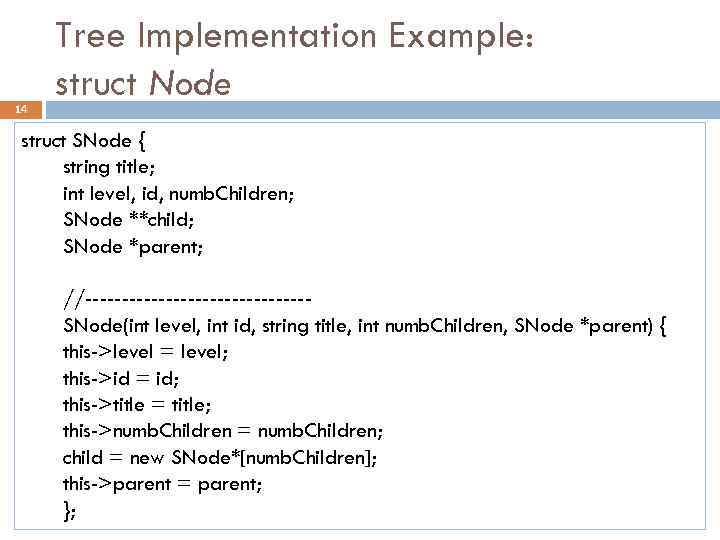14 Tree Implementation Example: struct Node struct SNode { string title; int level, id, numb. Children; SNode **child; SNode *parent; //---------------SNode(int level, int id, string title, int numb. Children, SNode *parent) { this->level = level; this->id = id; this->title = title; this->numb. Children = numb. Children; child = new SNode*[numb. Children]; this->parent = parent; };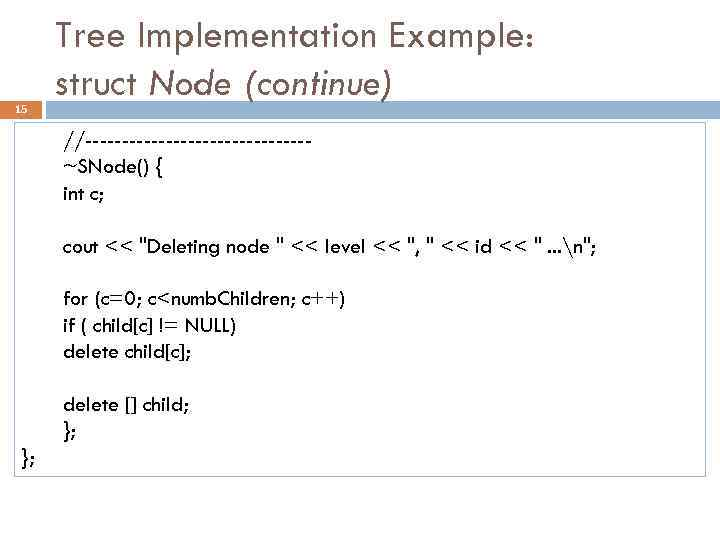15 Tree Implementation Example: struct Node (continue) //---------------~SNode() { int c; cout << "Deleting node " << level << ", " << id << ". . . n"; for (c=0; c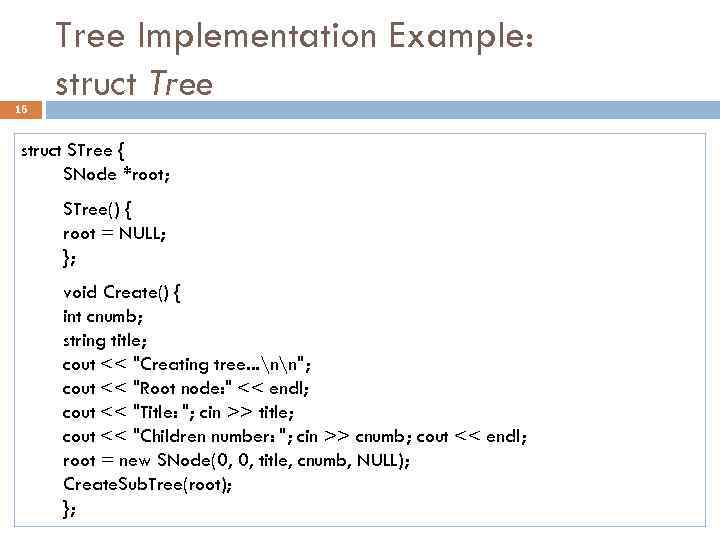16 Tree Implementation Example: struct Tree struct STree { SNode *root; STree() { root = NULL; }; void Create() { int cnumb; string title; cout << "Creating tree. . . nn"; cout << "Root node: " << endl; cout << "Title: "; cin >> title; cout << "Children number: "; cin >> cnumb; cout << endl; root = new SNode(0, 0, title, cnumb, NULL); Create. Sub. Tree(root); };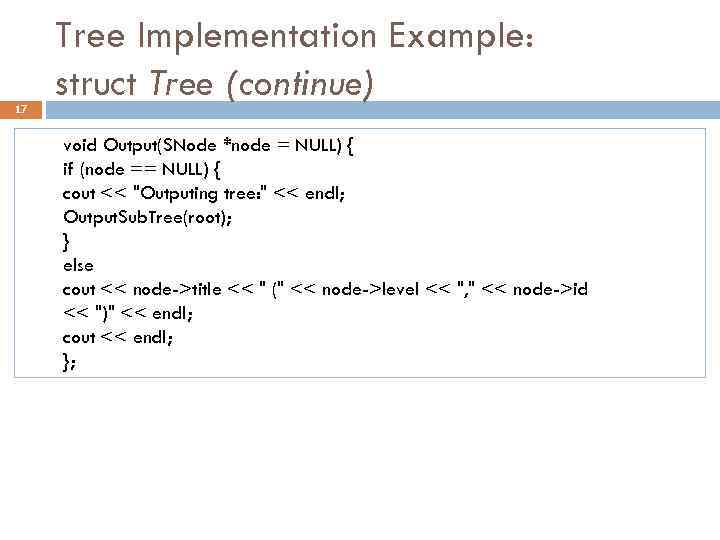17 Tree Implementation Example: struct Tree (continue) void Output(SNode *node = NULL) { if (node == NULL) { cout << "Outputing tree: " << endl; Output. Sub. Tree(root); } else cout << node->title << " (" << node->level << ", " << node->id << ")" << endl; cout << endl; };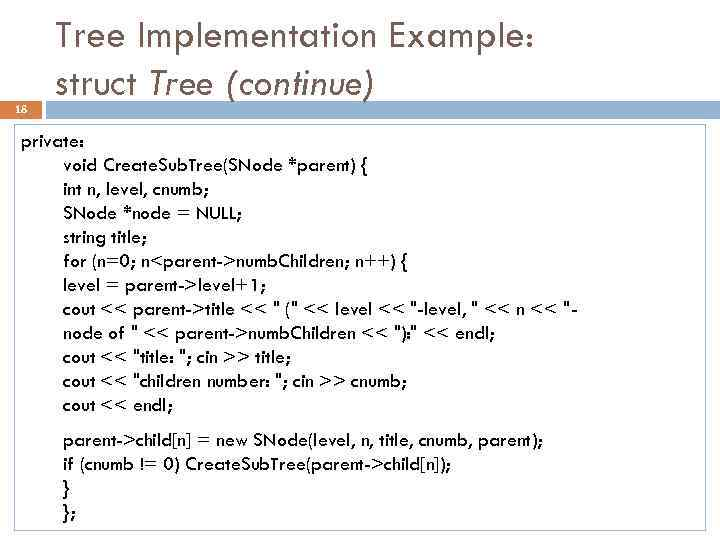18 Tree Implementation Example: struct Tree (continue) private: void Create. Sub. Tree(SNode *parent) { int n, level, cnumb; SNode *node = NULL; string title; for (n=0; nnumb. Children; n++) { level = parent->level+1; cout << parent->title << " (" << level << "-level, " << n << "node of " << parent->numb. Children << "): " << endl; cout << "title: "; cin >> title; cout << "children number: "; cin >> cnumb; cout << endl; parent->child[n] = new SNode(level, n, title, cnumb, parent); if (cnumb != 0) Create. Sub. Tree(parent->child[n]); } };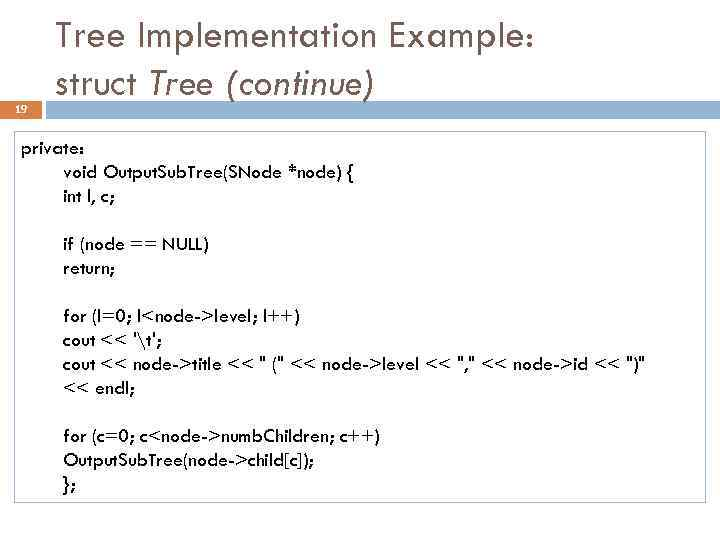19 Tree Implementation Example: struct Tree (continue) private: void Output. Sub. Tree(SNode *node) { int l, c; if (node == NULL) return; for (l=0; llevel; l++) cout << 't'; cout << node->title << " (" << node->level << ", " << node->id << ")" << endl; for (c=0; cnumb. Children; c++) Output. Sub. Tree(node->child[c]); };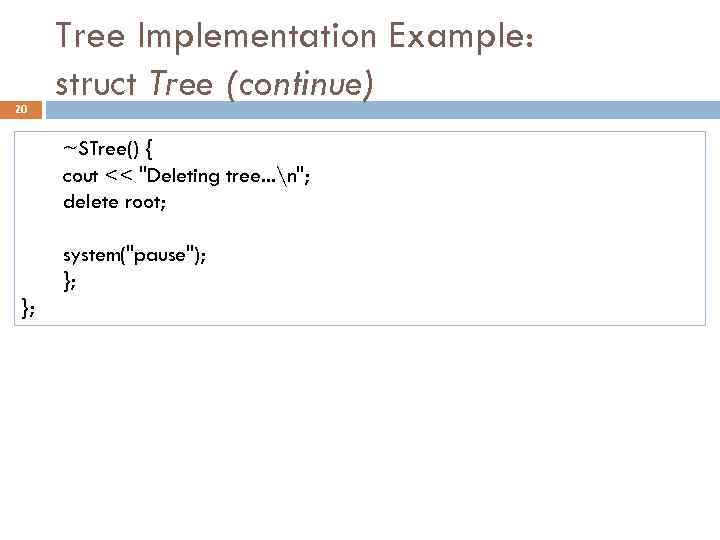20 Tree Implementation Example: struct Tree (continue) ~STree() { cout << "Deleting tree. . . n"; delete root; system("pause"); };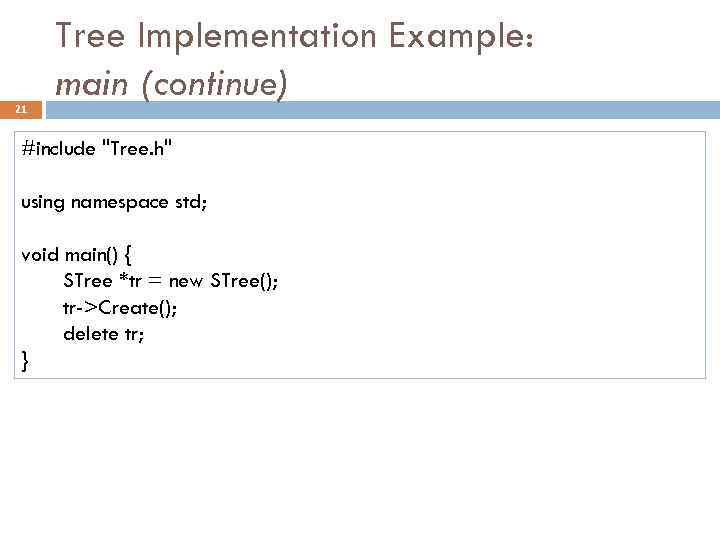21 Tree Implementation Example: main (continue) #include "Tree. h" using namespace std; void main() { STree *tr = new STree(); tr->Create(); delete tr; }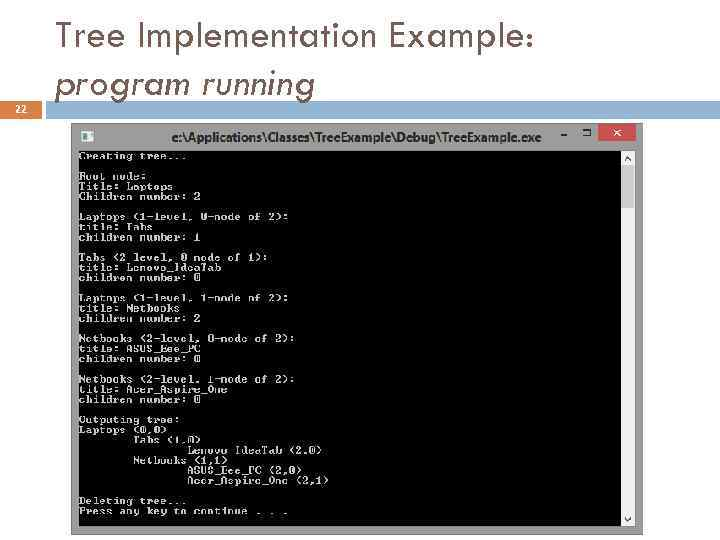22 Tree Implementation Example: program running